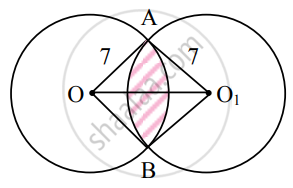Advertisement Remove all ads

# Answer the following : Two circles each of radius 7 cm, intersect each other. The distance between their centres is 72 cm. Find the area common to both the circles. - Mathematics and Statistics

Sum

Answer the following :

Two circles each of radius 7 cm, intersect each other. The distance between their centres is 7sqrt(2) cm. Find the area common to both the circles.

Advertisement Remove all ads

#### Solution

Let O and O1 be the centres of two circles intersecting each other at A and B.

Then OA = OB = O1A = O1B = 7 cm

and OO12 = 98  ...(i)

Since OA2 + O1A2 = 72 + 7

= 98

= OO12  ...[From (i)]

∴ m∠OAO1 = 90°

∴ m∠OAO1B is a square.

m∠AOB = m∠AO1B = 90°

= (90 xx pi/180)^"c"

= (pi/2)^"c"Now, A(sector OAB) = 1/2"r"^2theta

= 1/2 xx 7^2 xx pi/2

= (49pi)/4"sq.cm"

and A(sector O1AB) = 1/2"r"^2theta

= 1/2 xx 7^2 xx pi/2

= (49pi)/4"sq.cm"

A(∠OAO1B) = (side)2 = (7)2 = 49 sq.cm

∴ Required area = area of shaded portion

= A(sector OAB) + A(sector O1AB) – A(∠OAO1B)

= (49pi)/4 + (49pi)/4 - 49

= (49pi)/2 - 49

= 49(pi/2 - 1)"sq.cm"

Concept: Area of a Sector of a Circle
Is there an error in this question or solution?
Advertisement Remove all ads

#### APPEARS IN

Balbharati Mathematics and Statistics 1 (Arts and Science) 11th Standard Maharashtra State Board
Chapter 1 Angle and its Measurement
Miscellaneous Exercise 1 | Q 2. II (02) | Page 12
Advertisement Remove all ads
Advertisement Remove all ads
Share
Notifications

View all notifications

Forgot password?